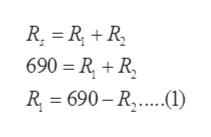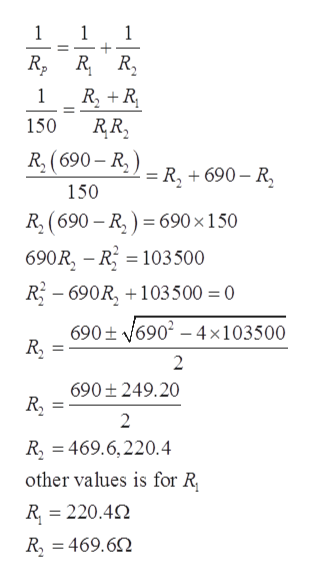# Two resistors connected in series have an equivalent resistanceof 690 Ω. When they are connected in parallel, their equivalent resistance is 150 Ω. Find the resistance of each resistor.

Question
1 views

Two resistors connected in series have an equivalent resistance
of 690 Ω. When they are connected in parallel, their equivalent resistance is 150 Ω. Find the resistance of each resistor.

check_circle

Step 1

Given:

Step 2

Equivalent resistance for series combination can be calculated ashelp_outlineImage TranscriptioncloseR, R + R, 690 = R, + R, R = 690 – R,..(1) fullscreen
Step 3

Equivalent resistance for parallel co...help_outlineImage Transcriptionclose1 1 1 R, R R, R, + R RR, 150 R, (690 – R, ) = R, + 690 – R, 150 R, (690 – R, ) = 690 ×150 %3D 690R, – R = 103500 R - 690R, +103500 = 0 690+ v6902 – 4×103500 R, 690 ± 249.20 R, R, = 469.6,220.4 other values is for R, R = 220.4N R, = 469.62 - | fullscreen

### Want to see the full answer?

See Solution

#### Want to see this answer and more?

Solutions are written by subject experts who are available 24/7. Questions are typically answered within 1 hour.*

See Solution
*Response times may vary by subject and question.
Tagged in

### Current Electricity